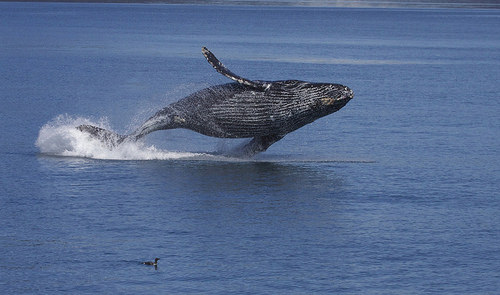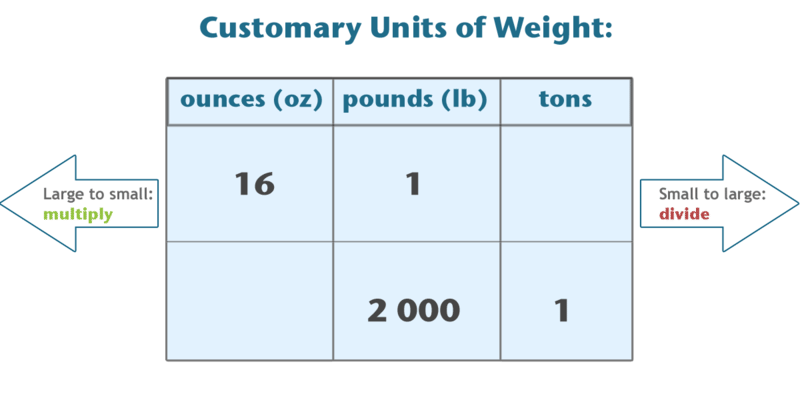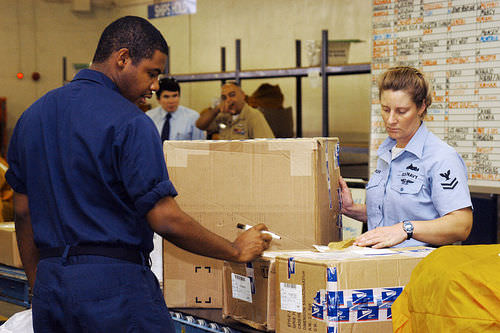# 6.2.4: Convert Between Ounces Pounds and Tons

•• Contributed by CK12
• CK12Figure $$\PageIndex{1}$$

While reading a book about marine animals, Sarah learns that the average humpback whale can weigh 40 tons. Yesterday, she learned that a polar bear weighs about 1000 pounds. How many polar bears will equal the weight of a humpback whale?

In this concept, you will learn about customary units of weight.

## Identifying Equal Customary Units of Weight

The United States uses the customary system of measurement. Measurements of length consist of units like inches, feet, yards and miles. The metric system is another system of measurement that is used in science and in most other countries.

Weight describes the heaviness or the way a mass of something, or someone, feels due to gravity. The customary units for measuring weight are ounces, pounds and tons.

Here are the customary units of weight from smallest to largest.

1. Ounce
2. Pound
3. Ton

When working with measures of weight, you can compare the equivalence of a small unit to a larger one. Remember, the word equivalent refers to something being equal to something else. Here are the customary units of equivalence.Figure $$\PageIndex{2}$$

You can find equivalent measures for each unit by using the information in the table. Convert from a larger unit to a smaller unit by multiplying. Convert from a smaller unit to a larger unit by dividing.

Use the information in the table to convert customary units of weight.

3 pounds = _____ ounces

First, figure out if you need to multiply or divide. Check the units. A pound is larger than an ounce, so you are going to multiply.

Then, check the table for the unit equivalence.

1 pound = 16 ounces

Finally, multiply the number of pounds times 16 to get the total number of ounces.

3 × 16 = 48

3 pounds is equivalent to 48 ounces.

Here is another conversion problem.

6,200 pounds = _____ tons

First, figure out if you need to multiply or divide. Check the units. You are converting a smaller unit to a larger unit, so divide.

Then, check the table for the unit equivalence.

2,000 pounds = 1 ton

Finally, divide the number of pounds by 2,000.

6,200 ÷ 2,000 = 3.1

6,200 pounds is equivalent to 3.1 tons.

Notice that the unit equivalent of 6,200 pounds to tons is not a whole number. Units of weight can be written as a fraction, a decimal, or with a remainder. A tenth of a ton is equal to 200 pounds.

Fractional answer: $$6,200\,÷\,2,000\,=\frac{6,200}{2,000}=3\frac{200}{2,000}=3\frac{1}{10}tons$$

Decimal answer: $$6,200\,÷\,2,000\,=\,3.1 tons$$

Remainder answer: $$6,200\,÷\,2,000\,=\,3\,R\,200\,=3\,tons\,200\,lbs$$

Examples

Example 1

Earlier, you were given a problem about Sarah and the animals.

Sarah knows that a polar bear weighs about 1000 pounds and a humpback whale weighs about 40 tons. Convert 40 tons to pounds to find the number of polar bears it would take to equal the weight of a humpback whale.

40 tons = _____ pounds

First, check if you will multiply or divide. A ton is larger than a pound. Multiply when converting from a larger to a smaller unit.

Then, find the unit equivalence.

2,000 pounds = 1 ton

Next, multiply the number of tons by the unit equivalence.

40 times 2,000 = 80,000

Finally, divide the weight of the whale, in pounds, by the weight of the polar bear, in pounds.

80,000 pounds divided by 1000 pounds = 80

Sarah calculates that it will take about 80 polar bears to equal the weight of a humpback whale!

Example 2

Convert the customary unit of weight.

A male African elephant usually weighs between 6 and 8 tons. If this the range of his weight in tons, what is the range of his weight in pounds?

First, decide if this conversion requires multiplication or division. Converting a larger unit to a smaller unit requires multiplication.

6 tons = _____ pounds

8 tons = _____ pounds

Then, use the unit equivalence.

2,000 pounds = 1 ton

Finally, multiply the number of tons by 2000 to find the range in tons.

6 × 2,000 = 12,000

8 × 2,000 = 16,000

The range of weight of a male African elephant is between 12,000 and 16,000 pounds.

Example 3

Convert the customary unit of weight: 5 tons = ____ pounds.

First, check if you will multiply or divide. Multiply when converting a larger unit to a smaller unit.

Then, find the unit equivalence.

1 ton = 2,000 pounds

Finally, multiply 5 by 2,000.

5 × 2,000 = 16,000

The answer is 5 tons equals 16,000 pounds.

Example 4

Convert the customary unit of weight: 32 ounces = ____ pounds.

First, check if you will multiply or divide. Divide when converting a smaller unit to a larger unit.

Then, find the unit equivalence.

16 ounces = 1 pound

Finally, divide 32 ounces by 16.

32 ÷ 16 = 2

The answer is 32 ounces equals 2 pounds.

Example 5

Convert the customary unit of weight: 4,500 pounds = ____ tons.

First, check if you will multiply or divide. Divide when converting a smaller unit to a larger unit.

Then, find the unit equivalence.

2,000 pounds = 1 ton.

Finally, divide 4,500 by 2,000.

4,500 ÷ 2,000 = 2.25

The answer is 4,500 pounds equals 2.25 tons.

Review

Convert each customary unit of weight.

1. 32 ounces = ____ pounds
2. 6 pounds = ____ ounces
3. 5.5 pounds = ____ ounces
4. 60 ounces = ____ pounds
5. 9 pounds = ____ ounces
6. 4000 pounds = ____ tons
7. 4 tons = ____ pounds
8. 3.5 tons = ____ pounds
9. 6500 pounds = ____ tons
10. 7.25 tons = ____ pounds
11. 15 pounds = ____ ounces
12. 25 tons = _____ pounds
13. 64 ounces = ____pounds
14. 80 ounces = ____ pounds
15. 5 tons = _____ pounds

To see the Review answers, open this PDF file and look for section 7.13.

## Ounces to MailFigure $$\PageIndex{3}$$

Have you ever wondered why you’d need to be able to convert and understand equivalent units of weight? Why would it matter whether something was calculated from ounces to pounds, or from pound to tons, or from tons to pounds, or from pounds back to ounces? Well, the reason’s as simple as mailing a package with the United States Postal Service!

### Why It Matters

Since January 2013, when you mail a package, the post office charges $1.95 for the first 3 ounces of weight. Each additional ounce thereafter costs$0.17. You need to understand pounds and ounces if you are planning to mail a package that weighs 2 pounds.Figure $$\PageIndex{4}$$

How would you figure out the cost of this 2-pound package? Well, you know that there are 16 ounces in 1 pound. The package weighs 2 pounds, which is equivalent to 32 ounces. Given that the first 3 ounces cost $1.95 and each additional ounce is$0.17, we can figure out the cost for 32 ounces of package:

$1.95+$0.17(32−3)=$1.95+$0.17(29)=$1.95+$4.93=\$6.88

See how the post office works: http://www.youtube.com/watch?v=KYFtalTNzKk

## Explore More

Visit the following website to play a variety of interactive measurement games.

http://www.aasd.k12.wi.us/staff/boldtkatherine/MathResources3-6/Math_Measurement.htm